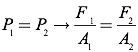# Pascal's Principle

When a force is applied to a contained, incompressible fluid, the pressure increases equally in all directions throughout the fluid. This fundamental characteristic of fluids provides the foundation for hydraulic systems found in barbershop chairs, construction equipment, and the brakes in your car.Because the force applied to the contained fluid is distributed throughout the system, you can multiply the applied force through this application of Pascal’s Principle in the following manner. Assume you have a closed container filled with an incompressible fluid with two pistons of differing areas, A1 and A2. If you apply a force, F1, to the piston of area A1, you create a pressure in the fluid which you can call P1.Similarly, the pressure at the second piston, P2, must be equal to F2 divided by the area of the second piston, A2.Since the pressure is transmitted equally throughout the fluid in all directions according to Pascal’s Principle, P1 must equal P2.Rearranging to solve for F2, you find that F2 is increased by the ratio of the areas A2 over A1.Therefore, you have effectively increased the applied force F1. Of course, the law of conservation of energy cannot be violated, so the work done on the system must balance the work done by the system. In the hydraulic lift diagram shown on the previous page, the distance over which F1 is applied will be greater than the distance over which F2 is applied, by the exact same ratio as the force multiplier!

Question: A barber raises his customer’s chair by applying a force of 150N to a hydraulic piston of area 0.01 m2. If the chair is attached to a piston of area 0.1 m2, how massive a customer can the chair raise? Assume the chair itself has a mass of 5 kg.Answer: To solve this problem, first determine the force applied to the larger piston.If the maximum force on the chair is 1500N, you can now determine the maximum mass which can be lifted by recognizing that the force that must be overcome to lift the customer is the force of gravity, therefore the applied force on the customer must equal the force of gravity on the customer.If the chair has a mass of 5 kilograms, the maximum mass of a customer in the chair must be 148 kg.

Question: A hydraulic system is used to lift a 2000-kg vehicle in an auto garage. If the vehicle sits on a piston of area 0.5 square meter, and a force is applied to a piston of area 0.03 square meters, what is the minimum force that must be applied to lift the vehicle?

Answer: